• 图像分类任务
• 收集训练集与测试集
• 支持向量机分类基本原理
• 特征选择与提取
• SVM 进行图像分类
• 分类结果评价

# 1. 图像分类任务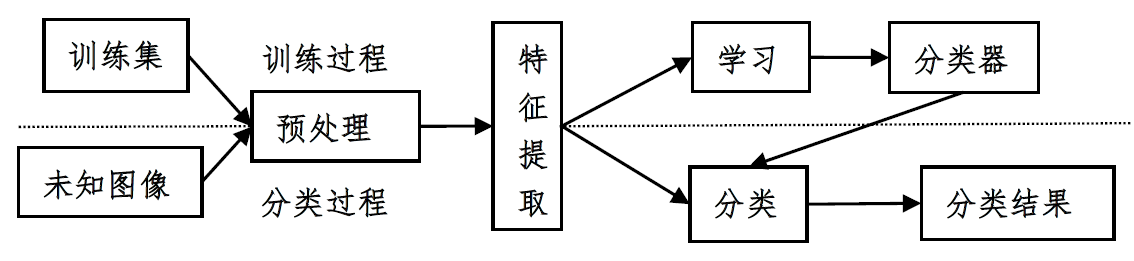# 2. 收集训练集与测试集

cifar10图像集是常用的图像分类数据集，由多伦多大学的Geoffrey Hinton教授等人收集整理，包含了60000张大小为32*32的彩色图像，其中50000张作为训练数据，10000张用于测试数据。50000张训练图片分为5个子集，分别命名为data_batch_1~5。每个子集都包含正好10000张图片，cifar10包含的图片类别如下图所示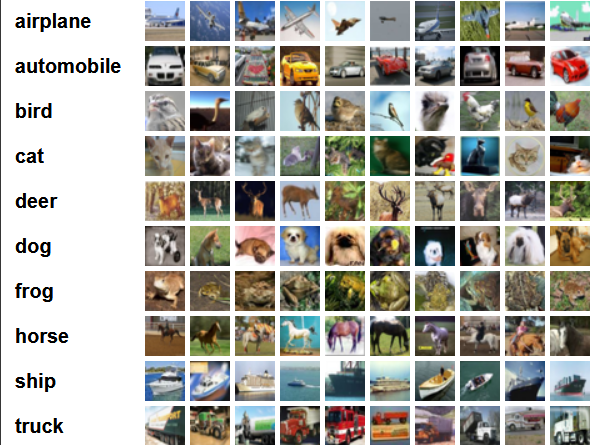# 3. 支持向量机分类基本原理

SVM是以最优化理论为基础来处理机器学习的新方式。它的提出主要是用来解决两类分类的问题，在两类中寻找一个最优超分平面将两类分开，来提高分类的正确率。

wxb=0  w ⋅ x − b = 0

(xi,yi),i=1,...,n,xϵRd,yϵ{+1,1} ( x i , y i ) , i = 1 , . . . , n , x ϵ R d , y ϵ { + 1 , − 1 }

yi[(w,xi)b]10,i=1,...,n y i [ ( w , x i ) − b ] − 1 ≥ 0 , i = 1 , . . . , n

## 3.1 线性可分情况

min:J(w,b,a)=12wTwi=1Nai[yi(wTxi+b)1] m i n : J ( w , b , a ) = 1 2 w T w − ∑ i = 1 N a i [ y i ( w T x i + b ) − 1 ]
yi(ωTixi+b)+1 y i ( ω i T x i + b ) ≥ + 1

Q(a)=j=1Nai12i=1Nj=1Naiajyiyj(xi,xj) Q ( a ) = ∑ j = 1 N a i − 1 2 ∑ i = 1 N ∑ j = 1 N a i a j y i y j ( x i , x j )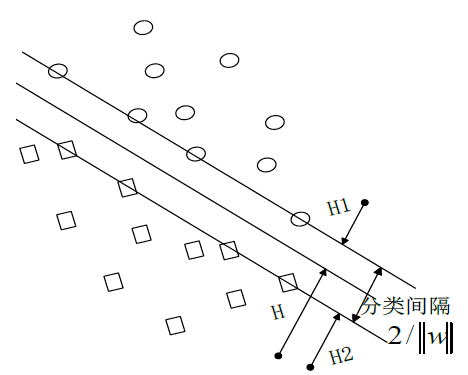## 3.2 非线性可分

max:Q(a)=j=1Nai12i=1Nj=1Naiajyiyj(xi,xj) m a x : Q ( a ) = ∑ j = 1 N a i − 1 2 ∑ i = 1 N ∑ j = 1 N a i a j y i y j ( x i , x j )
i=1Naiyi=0,ai0,i=1,2,...,N ∑ i = 1 N a i y i = 0 , a i ≥ 0 , i = 1 , 2 , . . . , N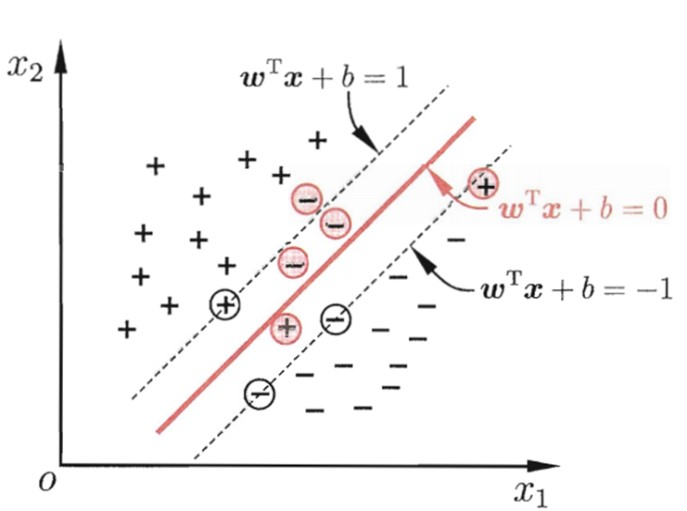• 多项式核函数： k(x,xi)=[(xxi)+1]q k ( x , x i ) = [ ( x ⋅ x i ) + 1 ] q $k(x,x_i )=[(x\cdot x_i )+1]^q$
• 基于径向基函数RBF核函数形式： k(x,xi)=exp|xxi|2/σ2 k ( x , x i ) = e x p ⁡ − | x − x i | 2 / σ 2 $k(x,x_i )=exp⁡{-|x-x_i |^2/σ^2}$
• Sigmoid核函数： k(x,xi)=tanh[v(xxi)+c] k ( x , x i ) = t a n h ⁡ [ v ( x ⋅ x i ) + c ] $k(x,x_i )=tanh⁡[v(x\cdot x_i )+c]$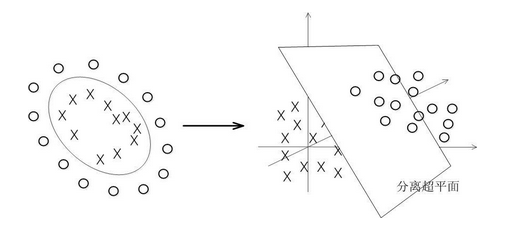SVM数据集形成的分类函数具有这样的性质：它是一组以支持向量为参数的非线性函数的线性组合。因此，分类函数的表达式仅和支持向量的数量有关，而独立于空间的维度。在处理高维输入空间的分类时，这种方法尤其有效。

## 3.3 一对一SVM(1-VS-1 SVMS)

fst(x)=wstϕ(x)+bst=i=svastiyik(xi,x)+bst f s t ( x ) = w s t ⋅ ϕ ( x ) + b s t = ∑ i = s v a i s t y i k ( x i , x ) + b s t

# 4. 特征选择与提取

## 4.1 方向梯度直方图

HOG特征描述子，它通过计算和统计图像局部区域的梯度方向直方图来构成特征，广泛应用于图像处理中进行物体检测。提取HOG特征包括以下几个步骤：

1) 归一化图像。首先把输入的彩色图像转灰度图像，然后对图像进行平方根Gamma压缩，从而达到归一化效果。这种压缩处理能够有效地降低图像局部的阴影和光照变化，从而提高HOG特征对于光照变化的鲁棒性。

2) 计算图像梯度。首先用一维离散微分模版[-1,0,1]及其转置分别对归一化后的图像进行卷积运算，得到水平方向的梯度分量以及垂直方向的梯度分量。然后根据当前像素点的水平梯度和垂直梯度，得到当前像素点的梯度幅值和梯度方向。公式如下：

Gx(x,y)=H(x+1,y)H(x1,y) G x ( x , y ) = H ( x + 1 , y ) − H ( x − 1 , y )
Gy(x,y)=H(x,y+1)H(x,y1) G y ( x , y ) = H ( x , y + 1 ) − H ( x , y − 1 )
G(x,y)=Gx(x,y)2+Gy(x,y)2 G ( x , y ) = G x ( x , y ) 2 + G y ( x , y ) 2
α(x,y)=tan1((Gy(x,y))/(Gx(x,y))) α ( x , y ) = t a n − 1 ( ( G y ( x , y ) ) / ( G x ( x , y ) ) )

3) 为每个细胞单元构建梯度方向直方图。首先把尺寸为64×128的图像分为8×16个cell，即是每个cell为8×8个像素。然后把梯度方向限定在[0, π]，并将梯度方向平均分为9个区间（bin），每个区间20度。最后对cell内每个像素用梯度方向在直方图中进行加权投影，也就是说cell中的每个像素点都根据该像素点的梯度幅值为某个方向的bin进行投票，这样就可以得到这个cell的梯度方向直方图，也就是该cell对应的9维特征向量。

4) 把细胞单元组合成大的块（block），块内归一化梯度直方图。把相邻的2×2个cell形成一个block，这样每个block就对应着36维的特征向量。由于局部光照的变化以及前景和背景对比度的变化，使得梯度强度的变化范围非常大。为了进一步消除光照的影响，最后对block内的36维特征向量进行归一化。公式如下：

V=vv22+ε2 V = v ‖ v ‖ 2 2 + ε 2

5) 收集HOG特征。如图1所示，采用滑动窗口的方法，用block对样本图像进行扫描，扫描步长为一个cell，所以block之间其实是有重叠的。最后把所有归一化后的block特征串联起来就得到3780维特征向量。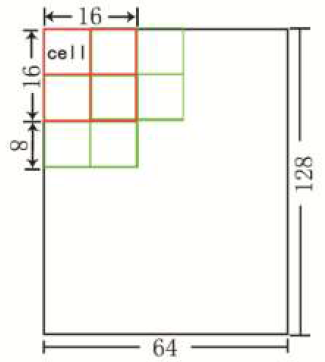## 4.2 灰度共生矩阵

p(i,j,d,θ)=[(x,y),(x+Dx,y+Dx)|f(x,y)=i,f(x+Dx,y+Dy)=j] p ( i , j , d , θ ) = [ ( x , y ) , ( x + D x , y + D x ) | f ( x , y ) = i , f ( x + D x , y + D y ) = j ]

ASM=i=0L1j=0L1[p(i,j,d,θ)] A S M = ∑ i = 0 L − 1 ∑ j = 0 L − 1 [ p ( i , j , d , θ ) ]
ENT=i=0L1j=0L1p(i,j,d,θ)Inp(i,j,d,θ) E N T = ∑ i = 0 L − 1 ∑ j = 0 L − 1 p ( i , j , d , θ ) I n p ( i , j , d , θ )
ENT=i=0L1j=0L1(ij)2[p(i,j,d,θ)] E N T = ∑ i = 0 L − 1 ∑ j = 0 L − 1 ( i − j ) 2 [ p ( i , j , d , θ ) ]
ASM=i=0L1j=0L1p(i,j,d,θ)[1+(ij)2] A S M = ∑ i = 0 L − 1 ∑ j = 0 L − 1 p ( i , j , d , θ ) [ 1 + ( i − j ) 2 ]

# 6. 分类结果评价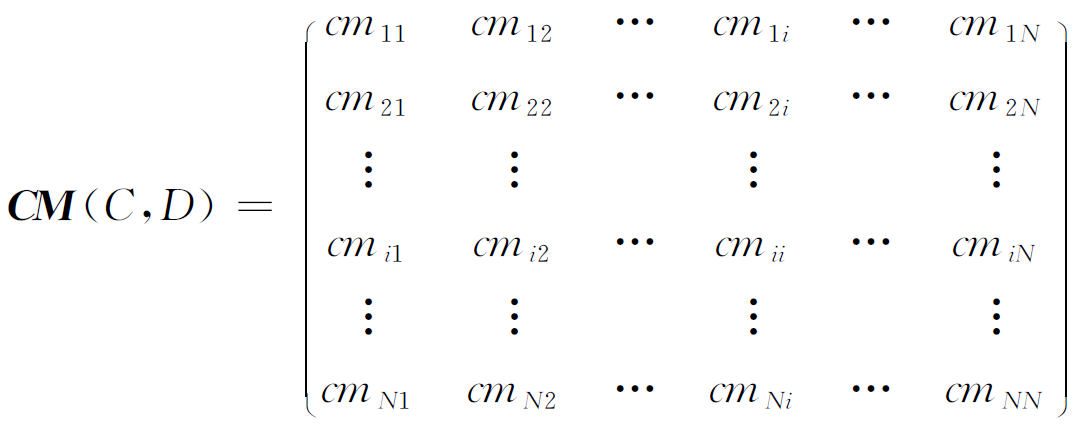(Acc)=×100% 准 确 率 ( A c c ) = 正 确 分 类 的 样 本 数 量 测 试 样 本 的 总 数 × 100 %

03-1706-07
07-254281
02-092万+
10-251618
06-141万+
05-261273
04-118万+
03-127057
03-242129
04-17
06-304万+
03-281万+
09-153299
04-084545
02-012297
11-014万+
03-26
10-21620
©️2020 CSDN 皮肤主题: Age of Ai 设计师:meimeiellie